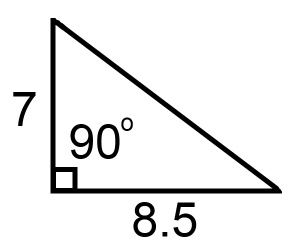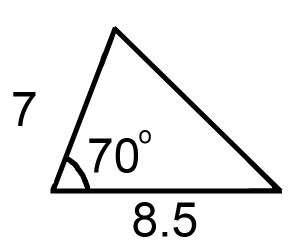#### You may also like### Doodles

Draw a 'doodle' - a closed intersecting curve drawn without taking pencil from paper. What can you prove about the intersections?### Russian Cubes

I want some cubes painted with three blue faces and three red faces. How many different cubes can be painted like that?### Areas and Ratios

Do you have enough information to work out the area of the shaded quadrilateral?

# Calculating with Cosines

### Why do this problem?

Many students are introduced to the cosine rule for the first time as a complicated formula they need to remember. In this problem, students are encouraged to seek a formula to work out the unknown side in a triangle. The suggested starting points can lead to deduction of the cosine rule, and by exploring it in several ways students will gain insight into where the formula comes from.

### Possible approach

Here is a way to approach this task with students who have not met the cosine rule.

"If I gave you this triangle, I'm sure you could work out the length of the missing side:""Here's a slightly different triangle. Do you think the missing side is longer or shorter? How do you know?""Is there a way of calculating the missing side if we know two of the sides and the angle in between them?"

Give students some time to work in small groups on trying to calculate the missing side for the particular example above, and then if they succeed, trying to generalise. Some suggested prompts you might offer:

"Could you add any lines to the diagram to create right angles?"

"Once you've created a right-angled triangle, what dimensions do you know? What can you work out?"

"If you drew the triangle on a coordinate grid, what would the coordinates of the vertices be?"

"If you know two points on a coordinate grid, how can you work out the distance between them?"

Once students have had a go at working out the missing side (either for this example or for the general case), offer them this worksheet which contains three starting points for working out the cosine rule.

You may wish to split the class into groups and give each group one method, before bringing the class together to demonstrate their methods to each other. Alternatively, all groups could work on all three methods and the session could finish with students evaluating the three methods and discussing which they prefer.

### Key questions

For Student 1's approach:

Can you make sense of each line of the method?
How can we express length x in terms of a, b and angle C?
Would the method work if angle C was obtuse?

For Student 2's approach:

Can you place any triangle on a coordinate grid in this way?
Would the method work if angle C was obtuse?

For Student 3's approach:

How can $\cos(180^{\circ}-C)$ be expressed in terms of $\cos C$?

### Possible extension

Challenge students to find a formula to work out the area of a triangle given two sides and the angle between them. This can then be used to prove the Sine Rule $(\frac{a}{\sin A} = \frac{b}{\sin B} = \frac{c}{\sin C})$.

### Possible support

Student 1's and Student 2's approach are more straightforward so students could just work on those two. By working in small groups, students can support each other to make sense of each method.